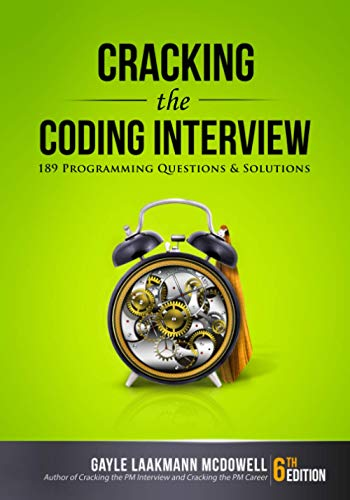# Power of 2

Question: How do you find out if an unsigned integer is a power of 2?

Answer: The trick here is to know that an unsigned integer which is a power of 2 has only one of its bits as 1. So a simple solution would be to loop through the bits and count the number of 1s.

There is another solution which uses bit manipulation.

isPower = (x !=0 && !(x & (x-1)))

x & (x-1) will always give you a 0 if x is a power of 2. !(x & (x-1)) should give us what we want but there is one corner case. If x is 0, then the second term alone would return true when the answer should be false. We need the check to make sure x is not 0.

Most interviewers would be happy with the first solution. The second solution might be quite hard to come up with on the spot unless of course you are a genius or you have read the solution before.

If you have any questions, please feel free to send me an email at [email protected]. If you have any interview questions which you feel would benefit others, I would love to hear about it.

If you're looking for some serious preparation for your interviews, I'd recommend this book written by a lead Google interviewer. It has 189 programming questions and solutions:## 2 Responses

1.satyam says:

int pow_of_two(unsigned num){

/*count the numbers of ones */

int i=0,count=0;
while(i!=31){
if(num&(1<<i)){
count++;
}
if(!(count%2 == 0)){
printf("Pow of 2");
}
else {
printf("Nah not power of 2");

}
}

/*not optimized but easy to understand*/

2.jagannath pattar says:

Easy way to find whether a number is power of 2.

/*
* A program to find whether a given number is a power of 2.
* [email protected]
*/

#include

void power_of_2(int num)
{
num = num & num-1;
if(num)
printf(“\nNumber is not power of 2\n”);
else
printf(“\nNumber is power of 2\n”);
}

main()
{
unsigned int num;
printf(“Enter the number: “);
scanf(“%d”, &num);
power_of_2(num);
}

XHTML: These are some of the tags you can use: `<a href=""> <b> <blockquote> <code> <em> <i> <strike> <strong>`